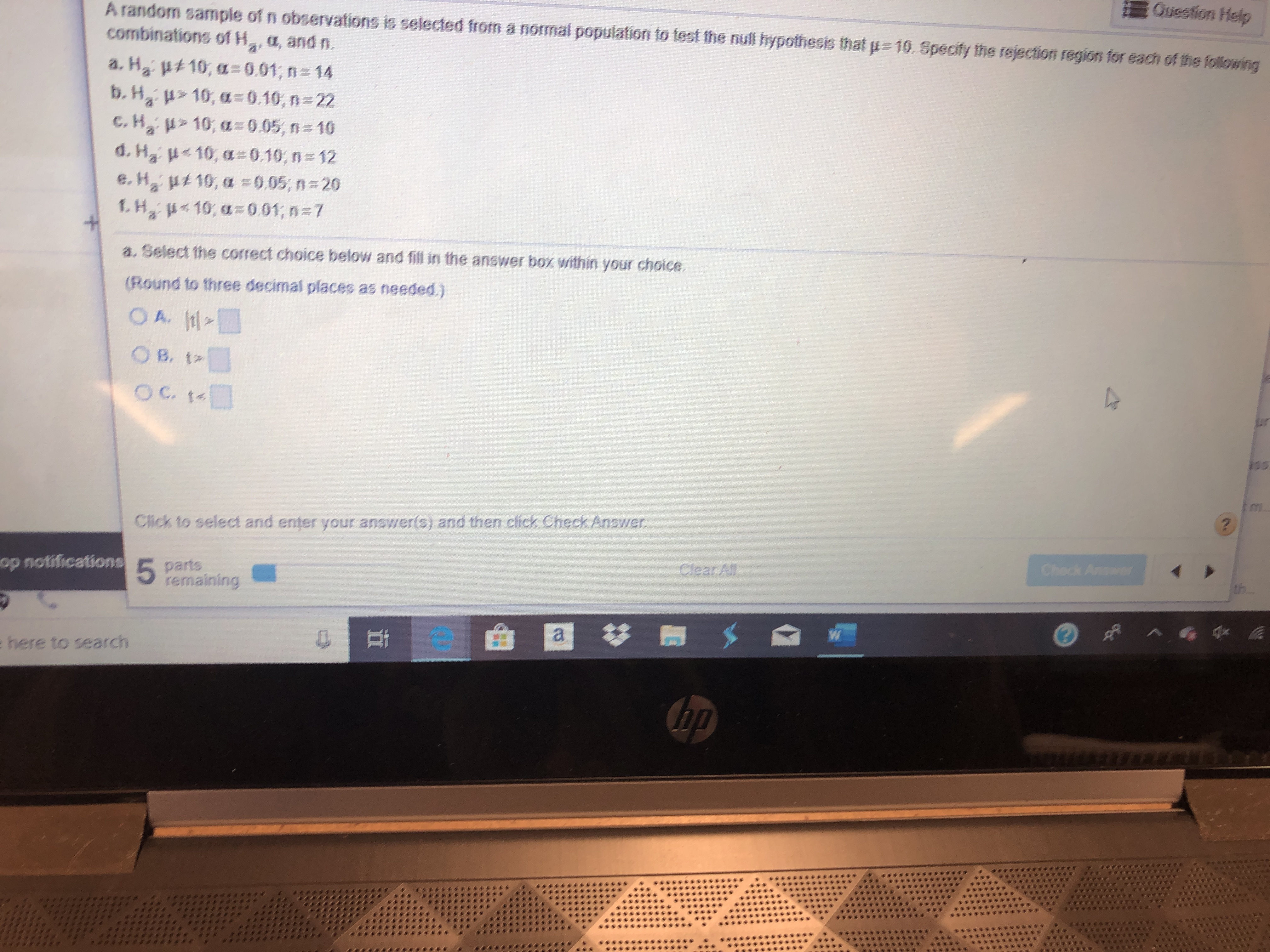# A random sample of n observafions is selected from a normal population to test the null hypothesis that p 10. Specify the rejection region for each of the followingQuestion Helpcombinations ofH, a, and n.aa. H2 ut 10, a0.01; n= 14b. H 10, a=0.10, n= 22C.H210, a 0.05, n 10d. H2 10, a=0.10, n=12e. H2 u10, a =0.05, n 20f.H 10, a=0.01; n=7a. Select the correct choice below and fill in the answer box within your choice.(Round to three decimal places as needed.)O A. tO B. t:OC t4inmClick to select and enter your answer(s) and then click Check Answer?op notifications5 partsremainingClear AllCheck Ansarae here to search41

Question
1 viewshelp_outlineImage TranscriptioncloseA random sample of n observafions is selected from a normal population to test the null hypothesis that p 10. Specify the rejection region for each of the following Question Help combinations ofH, a, and n. a a. H2 ut 10, a0.01; n= 14 b. H 10, a=0.10, n= 22 C.H210, a 0.05, n 10 d. H2 10, a=0.10, n=12 e. H2 u10, a =0.05, n 20 f.H 10, a=0.01; n=7 a. Select the correct choice below and fill in the answer box within your choice. (Round to three decimal places as needed.) O A. t O B. t: OC t4 in m Click to select and enter your answer(s) and then click Check Answer ? op notifications 5 parts remaining Clear All Check Ansar a e here to search 41 fullscreen
check_circle

Step 1

Note:

Hey there! Thank you for the question. Since you have posted several questions together, although the questions are given in parts a, b, c, etc., according t...

### Want to see the full answer?

See Solution

#### Want to see this answer and more?

Solutions are written by subject experts who are available 24/7. Questions are typically answered within 1 hour.*

See Solution
*Response times may vary by subject and question.
Tagged in

### Statistics